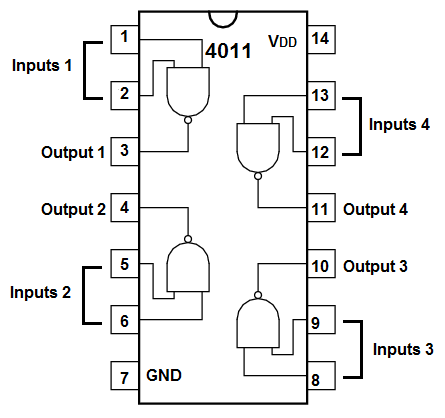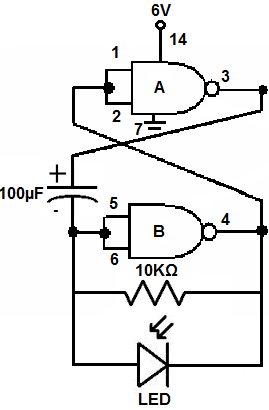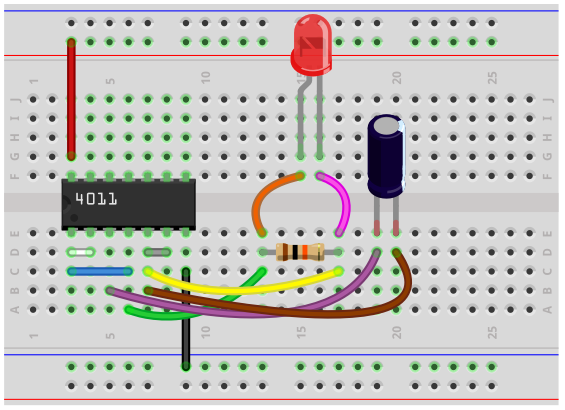﻿ How to Build an Astable Multivibrator Circuit with a 4011 NAND Gate Chip ﻿# How to Build an Astable Multivibrator Circuit with a 4011 NAND Gate ChipIn this circuit, we will show how to build an astable multivibrator circuit using a 4011 NAND gate chip with a capacitor and resistor.

By definition, an astable circuit is that one that oscillates between 2 states, HIGH and LOW, over and over and over again, indefinitely. It never holds one state. It constantly cycles from HIGH to LOW, HIGH to LOW, repeatedly. Therefore, it produces a square waveform signal as output or closely resembles it in some way.

To differentiate, a bistable circuit is one that is any 1 of 2 states, indefinitely. It will hold this stable state. This means the circuit will either be LOW or HIGH. However, if a trigger pulse of the correct polarity and amplitude is applied to the circuit, it can switch states. An astable circuit, on the other hand, does not hold any stable state but constantly switches between the 2 states of HIGH and LOW. Therefore, it's able to switch on and off components at a rate determined by the values of the components that are chosen.

So this circuit functions as an astable multivibrator. It's constantly going from ON to OFF, ON to OFF, repeatedly. An astable multivibrator is a multivibrator which has no stable state, so it's constantly switching from ON to OFF, indefinitely.

In this circuit, we will flash on an output device, an LED, over and over again, so that it flashes on and off, on and off. This is the essence of astable multivibrator functioning.

This can be used for many types of circuits including any where you want a device flashing on and off, on and off. Flashing LEDs are used in many types of electronic devices, including in cars, dashboards, printers, fax machines.

It can be used basically for any circuit in which you want a device turning on and off at repeatable intervals.

We will show how to build this circuit below. And we will show how we can modify the values of the capacitor and resistor to increase or decrease the flashing rate.

Components Needed

• 4011 NAND Gate Chip
• 10KΩ resistors
• 100µF electrolytic capacitor
• LED
• Second LED (optional)
• 470Ω resistors (optional)

The 4011 quad NAND gate chip can be obtained very cheaply from a number of online retailers for just a few cents. One place it can be obtained from is Tayda Electronics at the following link: Tayda Electronics- 4011 Quad 2-Input NAND Gate IC. However, it is a very popular chip and many electronics parts suppliers have them.

The pinout of the 4011 is shown below, so that you can see how to connect it in the circuit.Each NAND gate has input pins and 1 output pin.

The following chart shows NAND gate logic, which shows what output a NAND gate chip will produce for a set of given inputs.

 NAND Gate Logic Inputs Output 0 0 1 0 1 1 1 0 1 1 1 0

Since, in this circuit, we will tie together both inputs of the NAND gate, there are only 2 possible options that can be input into the circuit. Either both inputs will be 0, in which case the output is 1. Or both inputs will be 1, in which case the output is 0.

Any voltage below 1/2 the supply voltage will be interpreted as a 0. And any voltage greater than 1/2 of the supply voltage will be interpreted as a 1.

As far as the other components is concerned, the resistor and capacitor values aren't set in stone. The resistor and capacitor values of 10KΩ and 100µF are chosen so that it causes the LED to blink once a second. But you can choose any value, especially ones that are higher. It isn't recommended to choose lower values for them because then the rate of flashing will be so fast, it may not de detectable by the human eye. Therefore, 1 second should be seen as near the low end of the range for the frequency and shouldn't get much lower.

Current-limiting resistors for the LEDs are optional.

Also, a second LED is optional because this circuit can be modified to have 2 LEDs flashing alternatively like railway lights. We will show to connect this down below.

### Astable Multivibrator Circuit with a 4011 NAND Gate Chip

The astable multivibrator circuit we will build with a 4011 NAND gate chip is shown below.The breadboard circuit of the circuit above is shown below.So in order to power on the chip, we provide about 6V to VDD, pin 14, and connect VSS, pin 7, to ground. This establishes sufficient power for the chip and for our load, which is an LED, to operate. This circuit can actually operate on much less voltage; it can operate on as low as about 3.5V, so if you don't have the full 6V, it will operate on much less. We just use 6V for the LED and chip to operate on full brightness and power.

For this circuit, we use 2 of the 4 NAND gates from the 4011 chip.

The circuit works on the basis of feedback. The output for the 2 NAND gates are connected to the inputs of each of the NAND gates. So whatever is at the output for the NAND gates gets fed into the inputs of the other NAND gate.

So let's have the starting point as the capacitor, because the capacitor is the focal point of the circuit and is the most crucial element that allows for the astable capability.

When the circuit is turned on, the capacitor slowly charges up. It charges up and up and up. Once it hits the halfway point of the supply voltage, the capacitor discharges its charge through the resistor, turning on the output device, which is the LED. The LED turns on for the brief moment that the capacitor discharges through it.

When the capacitor charges up to its peak, a little above halfway of the supply voltage, it is HIGH, so the output of the second NAND gate is LOW. So a LOW signal is fed into the first NAND gate. This makes the output of the first NAND gate HIGH. Once the capacitor discharges through the resistor, it is LOW. This LOW signal creates a HIGH output at the second NAND gate. This HIGH signal is fed into the input of the first NAND gate, which creates a LOW output signal at the first NAND gate. The alternating states of the NAND gates occur according to the charging cycle of the capacitor. When the capacitor is below the midpoint of the supply voltage, the NAND gate outputs are in one state. When the capacitor is above the midpoint of the supply voltage, the NAND gate outputs switch states. So the capacitor charge and discharge cycles control the constant switching of states of the NAND gate.

Why the capacitor discharges at the point that it does is due to the fact that at above this value, the NAND interprets the input signal differently. So, again, the capacitor discharges at about at 1/2 the supply voltage. Below this point, the NAND interprets the signal as a LOW signal. Therefore, the output of the NAND gate is HIGH. When the capacitor reaches about midway or a little over midway the supply voltage, this point, the NAND gate interprets the signal as a HIGH signal and the output falls becomes LOW. Now at this stage, there is an electrical difference between the input and output. The output is LOW and the capacitor is now charged up to a decent amount of voltage. Since the NAND gate now becomes LOW, this electrical potential causes the capacitor to discharge through the resistor. This dumping of current which reaches over 60mA for a brief moment allows the LED to turn on. It's actually a pretty complex circuit.

The flashing rate of the LED is determined by the value of the resistor and capacitor. The resistor and capacitor flash at a rate equivalent to the formula, τ=RC. Since, in this circuit, we are using a 10KΩ resistor and a 100µF capacitor, this creates a time constant of τ=RC= (10KΩ)(100µF)= 1s. Therefore, the LED will flash on every second.

If you want to increase the frequency of the circuit, which is to make the time constant longer, you either increase the value of the capacitance or resistance or both. So, for example, if we changed the resistor to a 100KΩ resistor, while keeping the capacitor value unchanged, the LED would flash on every 10 seconds.

If you want to decrease the frequency of the circuit, which is to make the time constant shorter, you either decrease the capacitance or resistance or both. But keep in mind that if you decrease the frequency too much, the human eye may not be able to detect the change due to its speed. Therefore, it will just seem like the LED is permanently on. It switches so fast that the eye won't be able to detect when it's off. This is why using resistor and capacitor values that are too low are counterproductive for the sake of this circuit. You can lower it a little but if you do too much, it will seem as if it's not astable and it's just permanently connected on. So be aware of this.

So this is how this circuit works. But as with many circuits, there are modifications that we can do to enhance the circuit if needed.

One modification that we can do is to add a second LED to the circuit. A second LED is placed in parallel to the existing LED, only with the polarity switched. With this configuration, the circuit will light the LEDs alternately. So it will be like a railway lights. When one LED is on, the other is off and vice versa. The LEDs flash alternately. So this may be a configuration you want for a particular circuit.

One thing to know about this configuration is that, since there are LEDs in different polarity, current will bypass the resistor altogether. This is because the path of least resistance is through the anodes of each of the LEDs. Therefore, current avoids taking the resistor, since it offers substantially more resistance than the LEDs. Therefore, if you add a second LED in parallel in opposite polarity, you will see that the LEDs flash at a much faster rate than before. This is again is due to the bypassed resistor. If you are connecting the circuit with this configuration, the only way to change the flashing rate is due to the capacitor value. If you want to decrease the rate, you change the capacitor to a higher value. If you want to increase the rate, you change the capacitor to a lower value. You probably could add another resistor in series to both of the parallel components, but this complicates the circuit. So you just have to change the capacitor value. You could also remove the resistor in parallel completely because it serves no purpose.

For this circuit, you could add current-limiting 470Ω resistors to limit current to the LED. But it isn't absolutely necessary, just an extra protection measure.

And this is how an astable multivibrator circuit can be built with a 4011 NAND gate chip.

To see this circuit operating in real life, please see the video below.

Related Resources